# 2-D Arrays in C/C++

## 2-D Arrays in C/C++

In this article, we are going to discuss 2-D Arrays in C and C++ with Examples. Please read our previous article where we have already discussed the How to Increase the size of an array. At the end of this article, you will understand how to create, initialize, and access 2-Dimensional Arrays in C and C++.

In almost all programming languages like C, C++, Java, C#, etc., we can have multi-dimensional arrays. Basically, we use one dimension or two-dimensional Arrays. In very rare cases we go for 3-Dimensional Arrays. But the programming languages allow us to declare n-dimensional arrays. So, the commonly used is a 2-D array. The 2D array is mostly useful for implementing matrix or for tabular data. Now let’s see how to create a 2-Dimensional array in C and C++. There are three methods of creating a 2-D array so let us look at them.

##### First Method: Normal Declaration and Initialization of 2D array

The First method is the normal declaration of a 2-Dimensional Array along with the name of an array, data type of an array, and dimensions. For example, we want an array of sizes 3*4. So, we visualize that an array of size 3*4 will be created inside the main memory with 3 rows and 4 columns. The indices will be 0, 1, and 2 for rows and 0, 1, 2, and 3 for columns. For better understanding, please have a look at the below image.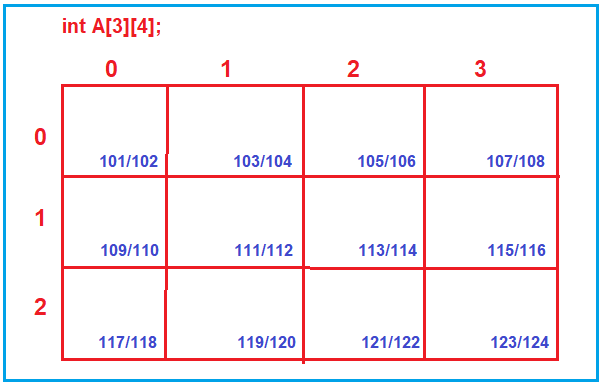We represent a 2-Dimensional array in a rectangular form i.e., like a box. But in reality, the memory allocation will be linear. For example, if you have a look at the above diagram, the addresses are contiguous. Let us assume that the first-byte address is 101 and let’s assume integer takes 2 bytes. So, the memory location address is 101/102, the next memory location address is 103/104, the next 105/106, and so on.

This means that in reality, the memory will be allocated like a single dimension array with 3*4=12 integers. But compilers allow us to access that 1-D array as a 2-D array with help of row number and column number indices. So, any location if you want to access, you can access it with the help row number and column number.

For example, you can access the second column of the first row using A; and you can set or get the value. So, the point that you need to remember is, we access the 2-Dimensional array with two indices that is row number and column number.

If you want to initialize a 2-Dimensional array, then you can directly mention the list of elements like 3 rows and 4 columns as shown below. The first row is having 4 elements {1,2,3,4} and the second row is having 4 elements {2,4,6,8} and third row is also having four elements {3,5,7,9}.

int A= {{1,2,3,4},{2,4,6,8},{3,5,7,9}};

Here, the array will be created inside the stack as it is just like a variable. There is no new operator use. If you use the new operator then only the array will be created in the heap. So, this is the first method of creating an array inside the Stack.

##### Second method: Using Array of Pointers

Now let’s look at the second method and see how we can create a 2-Dimensional array. We can take an array of pointers. So first create an array of size3 with an integer pointer as shown below.

int *A;

As this is an array i.e. a normal variable, so it will be created inside the stack. But this is not an array of integers. This is an array of integer pointers. We have just an array of three-pointers that are created in the stack as shown below.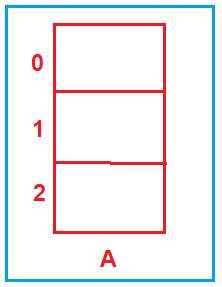We will create the 2-Dimensional array in the heap. What actually we want is, we want three arrays of each size 4 created in the help and each of these arrays will be pointed by the pointer from the stack. For better understanding, please have a look at the following image.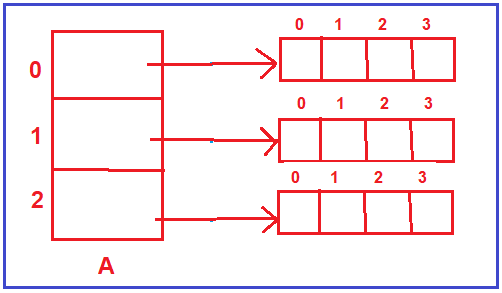As you can see in the above image, we have 3 arrays and we have pointer arrays pointing to those 3 arrays created in heap and together they form the same 2-Dimensional array i.e. 3 rows and 4 columns. This is the second method of creating the 2-D array.

Let’s see the syntax of how we can create these arrays.

int *A;
A = new int; //An array of size 4 will be created in the heap whose address will be store in A.
A = new int; //An array of size 4 will be created in the heap whose address will be store in A.
A = new int; //An array of size 4 will be created in the heap whose address will be store in A.

So, in this way, we created the memory in the heap for all those 3 arrays and the pointer array is present in the stack, and that pointer array stores the address of the arrays which are created in the heap.

Now even in this structure, we can access it just like a normal array. Suppose we want to store a value 15 in an array, then we can do the same by using the below syntax.

A=15; Here, the value 15 will be stored in the third column of the second array, For better understanding, please have a look at the below image.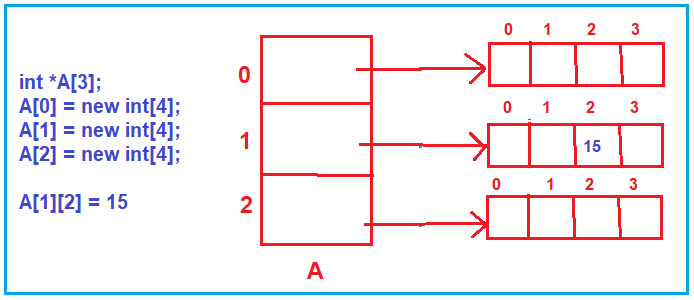##### Third method: Double Pointer

In the third method, we can take a double-pointer. Here almost everything is in the heap. The declaration of double-pointer is shown below.

int **A; Here, A is a double pointer and this is like a variable, there is no new operator, so this will be created inside the stack.

Now we need the following two arrays inside the heap and that should be pointed by the double-pointer variable which is inside the stack.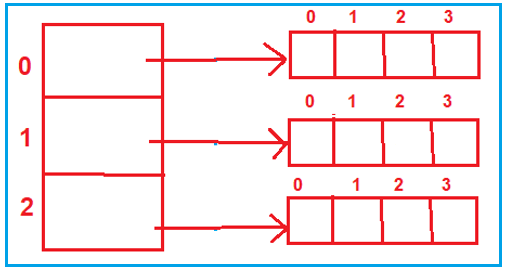For this, we need to use the below syntax.

A=new int*; it is created in heap i.e. array of the pointer is itself created in the heap. For better understanding, please have a look at the below image.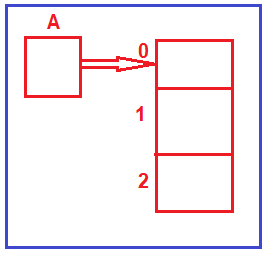Now we will prepare other arrays. We will create 3 more arrays and assign them to the previously created array pointer. How do create these three arrays? The same method that we used previously i.e., A=new int;  A =new int; A =new int; For better understanding, please have a look at the below image.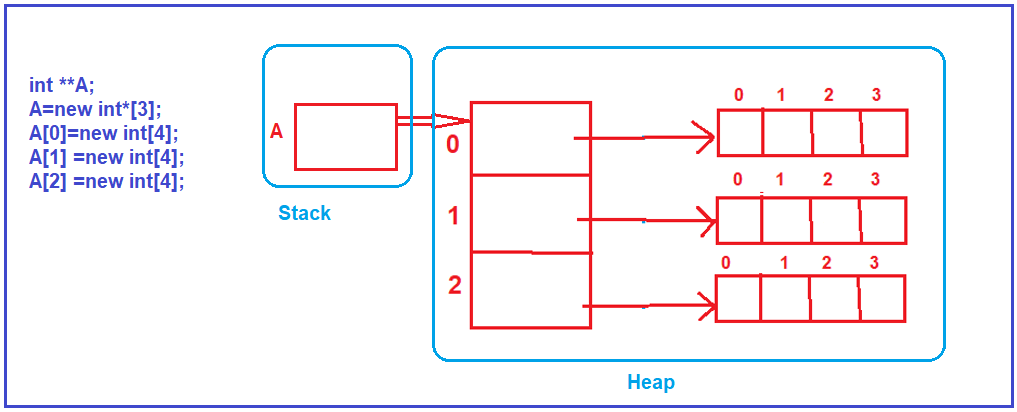Now one more thing, every time we used the new operator i.e. to create the memory in heap in C++ language. Then how it is done in the C language? Then you know very well that the malloc function is used for allocating memory in heap in C language.

##### How to access the 2Dimensional Array?

The 2-Dimensional arrays can be accessed by using nested two for loops. So, for accessing any of these arrays, we can use two nested for loops as shown below.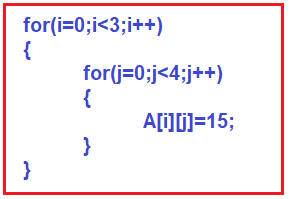This will help us to traverse through the 2-D array row by row. That’s all these are the few basic things that one must know about a 2-D array.

##### Example: Normal Method

The first method that we have shown you is we can create an array inside the stack. If you follow this method array will be created inside the stack. Suppose we want an array of 3 rows and 4 columns. So, this is a 2-D array of dimensions 3*4. If we want to initialize, then every row we should initialize. There are 3 rows and each row is having 4-4 elements because there are 4 columns. So, this is the way we can create a 2-D array, this will be created inside the stack. The following is the complete code.

```#include <stdio.h>
#include <stdlib.h>
int main()
{
int A={{1,2,3,4},{2,4,6,8},{1,3,5,7}};
int i,j;
for(i=0;i<3;i++)
{
for(j=0;j<4;j++)
{
printf("%d ",A[i][j]);
}
printf("\n");
}
return 0;
}
```

Output: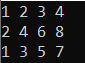##### Example: Using Array of Pointers

In this method we can take an array of pointers of size 3 i.e., *A, this is for rows, columns are not created, this array of the pointer is created inside the stack. Then we will create arrays in the heap and assign them to these arrays of pointers. The following is the complete code using C Language.

```#include <stdio.h>
#include <stdlib.h>
int main()
{
int *A;
int i,j;

A=(int *)malloc(4*sizeof(int));
A=(int *)malloc(4*sizeof(int));
A=(int *)malloc(4*sizeof(int));

//Assigning Array Elements
printf("Enter 3*4 Array Elements:");
printf("\n");
for(i=0;i<3;i++)
{
for(j=0;j<4;j++)
{
scanf("%d",&A[i][j]);
}
}

//Accessing Array Elements
printf("Entered 3*4 Array Elements Are: ");
printf("\n");
for(i=0;i<3;i++)
{
for(j=0;j<4;j++)
{
printf("%d ",A[i][j]);
}
printf("\n");
}
return 0;
}
```

Output: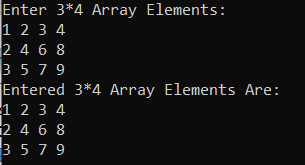##### Third Method: Double Pointer

In the third method, we have seen that we are using a double pointer here. i.e., int **A. The complete code is given below for C Language.

```#include <stdio.h>
#include <stdlib.h>
int main()
{
int **A;
int i,j;

A=(int **)malloc(3*sizeof(int *));

A=(int *)malloc(4*sizeof(int));
A=(int *)malloc(4*sizeof(int));
A=(int *)malloc(4*sizeof(int));

//Assigning Array Elements
printf("Enter 3*4 Array Elements:");
printf("\n");
for(i=0;i<3;i++)
{
for(j=0;j<4;j++)
{
scanf("%d",&A[i][j]);
}
}

//Accessing Array Elements
printf("Entered 3*4 Array Elements Are: ");
printf("\n");
for(i=0;i<3;i++)
{
for(j=0;j<4;j++)
{
printf("%d ",A[i][j]);
}
printf("\n");
}
return 0;
}
```

Output: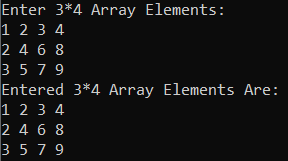In the next article, I am going to discuss Array Representation by Compiler. Here, in this article, I try to explain the basics of 2-Dimensional Array in C and C++. I hope you enjoy this 2-Dimensional Array in C and C++ article.